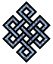#fail2ban bad ip database: ip 125.212.225.76

### | ip database | live view | stats | report | help | api key:

 ip: 125.212.225.76 hostname: 125.212.225.76 country:[VN] Vietnam first reported: 03.07.2015 18:07.57 GMT+0200 last reported: 18.03.2019 17:56.13 GMT+0200 time period: 1354d 48m 16s total reports: 18 reported by: 2 host(s) filter(s): ssh (17) ssh (1) tor exit node no badips.com db Lookup## port scan of '125.212.225.76':

[-hide]
```# Nmap 6.40 scan initiated Mon Mar 18 17:57:02 2019 as: /usr/bin/nmap -sU -sS -O 125.212.225.76
Nmap scan report for 125.212.225.76
Host is up (0.24s latency).
Not shown: 1980 filtered ports
PORT      STATE         SERVICE
21/tcp    closed        ftp
22/tcp    open          ssh
80/tcp    open          http
88/tcp    closed        kerberos-sec
1234/tcp  closed        hotline
1521/tcp  closed        oracle
2000/tcp  open          cisco-sccp
5080/tcp  closed        onscreen
5901/tcp  closed        vnc-1
8000/tcp  closed        http-alt
8001/tcp  closed        vcom-tunnel
8002/tcp  closed        teradataordbms
8021/tcp  closed        ftp-proxy
8080/tcp  closed        http-proxy
8081/tcp  open          blackice-icecap
8089/tcp  closed        unknown
8800/tcp  closed        sunwebadmin
8888/tcp  closed        sun-answerbook
8899/tcp  closed        ospf-lite
17946/udp open|filtered unknown
No exact OS matches for host (If you know what OS is running on it, see http://nmap.org/submit/ ).
TCP/IP fingerprint:
OS:SCAN(V=6.40%E=4%D=3/18%OT=22%CT=21%CU=%PV=N%G=Y%TM=5C8FD24C%P=x86_64-pc-
OS:linux-gnu)SEQ(SP=107%GCD=1%ISR=10A%TI=Z%CI=RI%TS=A)SEQ(SP=108%GCD=1%ISR=
OS:109%TI=Z%TS=D)SEQ(CI=RI%TS=B)SEQ(CI=RI)OPS(O1=M5B4ST11NW7%O2=M5B4ST11NW7
OS:%O3=M5B4NNT11NW7%O4=M5B4ST11NW7%O5=M5B4ST11NW7%O6=M5B4ST11)WIN(W1=7120%W
OS:2=7120%W3=7120%W4=7120%W5=7120%W6=7120)ECN(R=Y%DF=Y%TG=40%W=7210%O=M5B4N
OS:NSNW7%CC=Y%Q=)T1(R=Y%DF=Y%TG=40%S=O%A=S+%F=AS%RD=0%Q=)T2(R=N)T3(R=N)T4(R
OS:=Y%DF=Y%TG=40%W=0%S=A%A=Z%F=R%O=%RD=0%Q=)T5(R=Y%DF=Y%TG=40%W=0%S=Z%A=S+%
OS:F=AR%O=%RD=0%Q=)T6(R=Y%DF=Y%TG=40%W=0%S=A%A=Z%F=R%O=%RD=0%Q=)T7(R=N)U1(R
OS:=N)IE(R=Y%DFI=N%TG=40%CD=S)

OS detection performed. Please report any incorrect results at http://nmap.org/submit/ .
# Nmap done at Mon Mar 18 18:15:56 2019 -- 1 IP address (1 host up) scanned in 1134.94 seconds
```
```Σ = 66 | Δt = 0.0057351589202881s
```